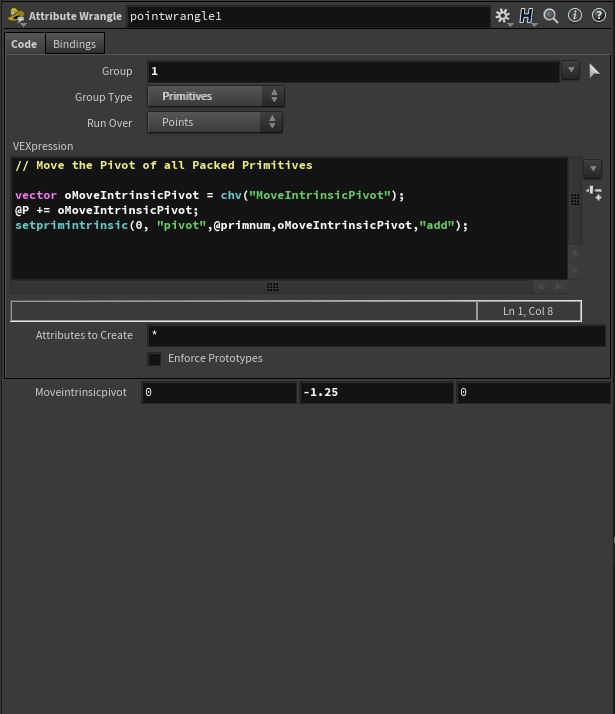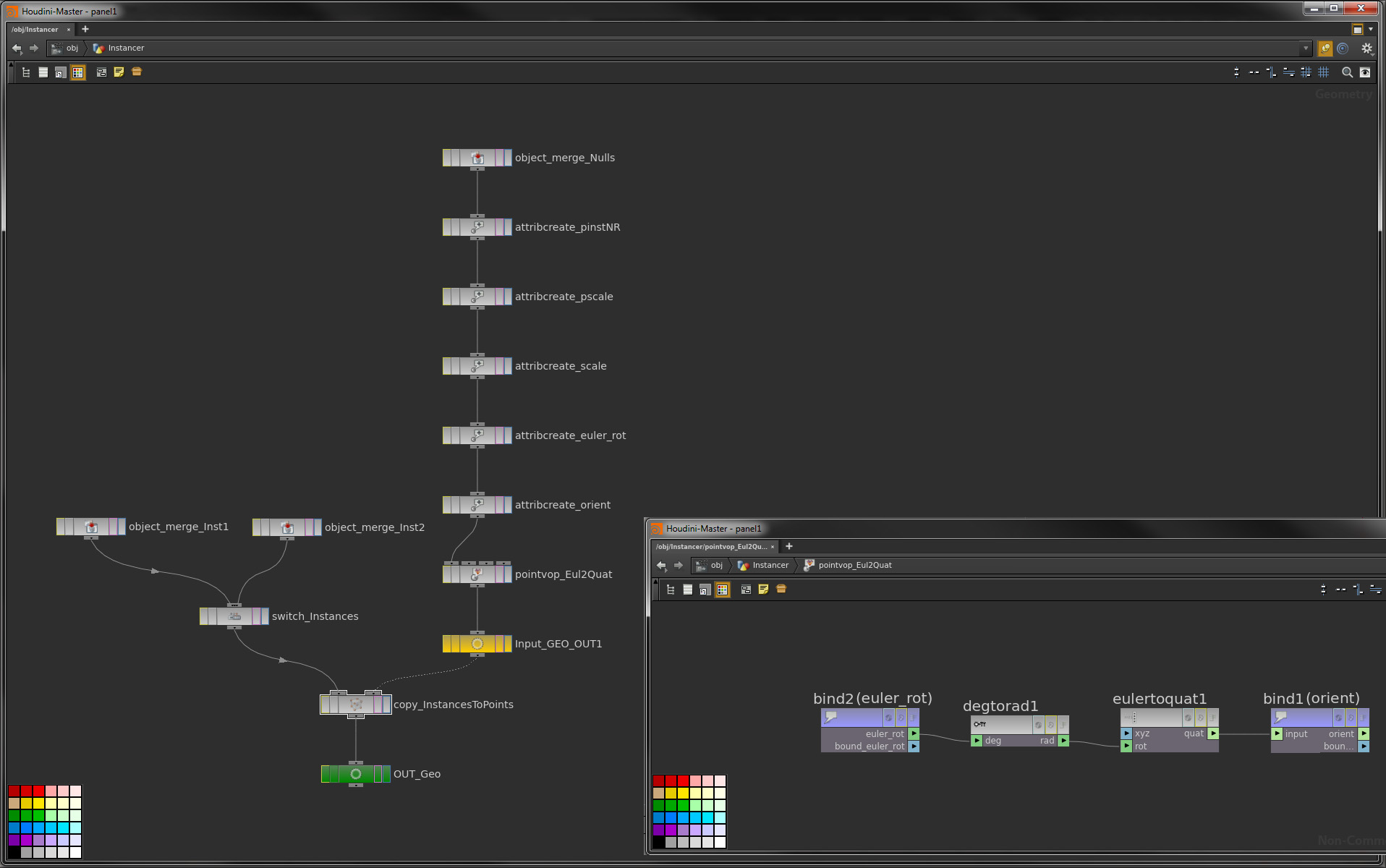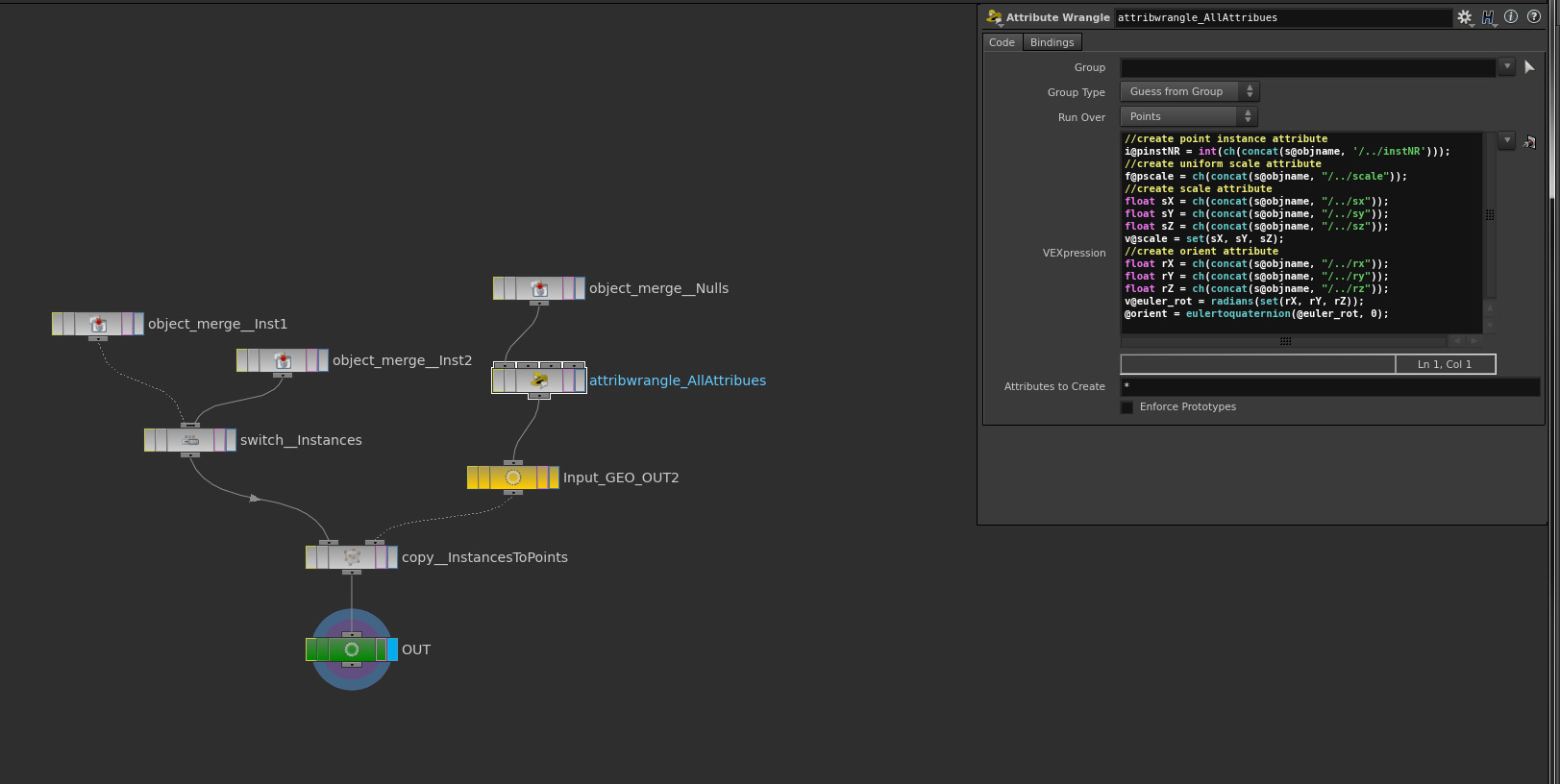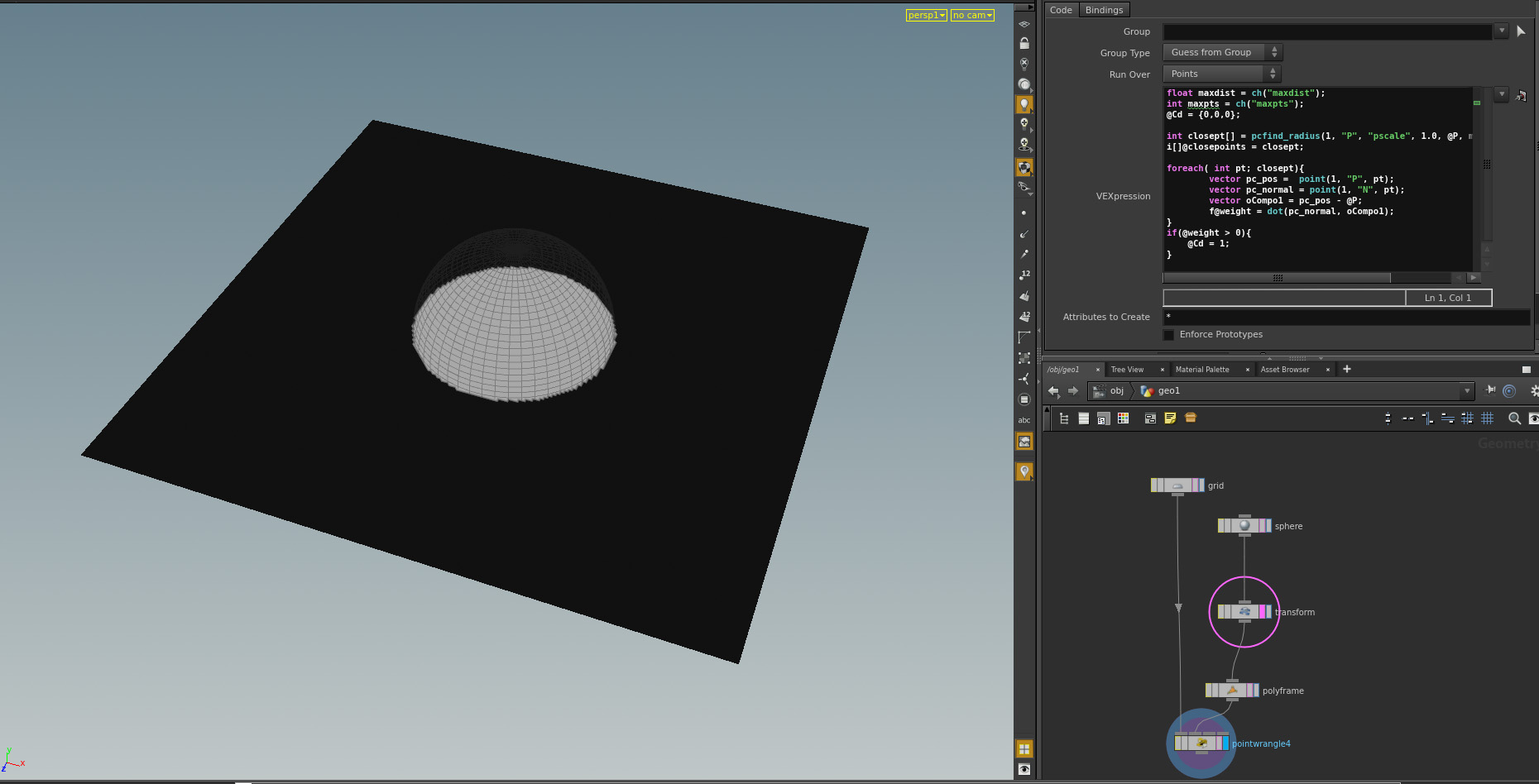VEX

Function of the Week: sample_direction_cone

Hey,

today I’ll establish my personal Node/Function of the week. This week it’s the vex function “sample_direction_cone”.

The help says: “Returns a unit vector, i.e. a vector of length 1, based on u. Given uniform random u pairs of values in [0,1), the returned unit vectors will be uniform random and continuous with respect to u on the surface of the unit sphere, in the area within maxangle of the direction indicated by center.”

So what to use this for? My usual cases are randomization of velocity vectors, ray cast operations or additive spread-transforms of scattered pieces. XSI operators will know this kind of function from the ICE function “randomize vector by cone” – This is the equal Houdini function.Here is an point wrangle example, that demonstrates the function in combination with an raycast function.

vector pos;
vector uvw;
int prim = intersect(1, @P, @dir*20, pos, uvw);
if(prim>=0){
@P = primuv(1, "P", prim, uvw);
}moving the privot of packed primitives

Today I had to move all pivots of an packed primitive object for an rbd simulation. If the centroid, center of mass or origin doesn’t fit your needs, maybe this could be interesting.

// move the pivot of all packed primitives

vector oMoveIntrinsicPivot = chv("MoveIntrinsicPivot");
@P += oMoveIntrinsicPivot;Instance/copy objects with nulls

Hey there,

when dealing with real commercial productions it is, at some point, necessary to place object instances by hand. There for you have four options in Softimage.

1. A hard copy of the object
2. A clone of the object
3. Put it in a Model and use an model instance
4. Use ICE to distribute instances in combination with Nulls that have an PPG with an integer attribute to detect the instance.Point four is the workflow I like the most, because it is super flexible. After the placement it is possible to change all attributes e.g. with a random size or position. Now the question is, how do I adapt my known workflow to Houdini? Exact the same way I do it in SI. Fist we need to create a null object with an integer attribute “instNR” for instance number, some instances and a geometry that holds the geometry.Lets dive into geometry level. First I have tried to build a setup the classical way. With maaaany attribute create sops.“Going from top to bottom you see that I create all attributes I need to transfer the data from the null objects: i@instNR, f@pscale, v@scale, v@euler_rot, 4f@orient. In the point vop I convert the euler rotation to a quaternion rotation. This is needed because the copy sop is using quaternions. With the path attribute of the object_merge sop we can access all attributes like this: ch(@objname + “/../instNR”). The only attribute we have to samp is the Instance Number. The syntax in the copy sop is like this: point(“../Input_GEO_OUT1”, \$PT, “pinstNR”, 0). The switch node can now access this attribute like this: stamp(“../copy_InstancesToPoints”, “pinstNR”, 0). This is all we need to get it working.

Much slicker is to do all attribute manipulation in an attribute wrangle.That’s all. Easy to setup and effective. The only thing to notice here is, that this is a copy NOT a instance workflow. To really instance objects I recommend this tutorial. https://vimeo.com/55292609 this workflows here can easily combined with this workflow. Here InstanceObjectsWithNulls you can download the example hip file.

Point in Volume (Feat VEX)

Hey!

In my last post I showed a simple “Point in Volume” VOP. Inspired from the Side FX VEX Webinar I ported this logic to VEX with an attribute wrangle.float maxdist = ch("maxdist");
int maxpts = ch("maxpts");
@Cd = {0,0,0};

int closept[] = pcfind_radius(1, "P", "pscale", 1.0, @P, maxdist, maxpts);
i[]@closepoints = closept;

foreach( int pt; closept){
vector pc_pos =  point(1, "P", pt);
vector pc_normal = point(1, "N", pt);
vector oCompo1 = pc_pos - @P;
f@weight = dot(pc_normal, oCompo1);
}
if(@weight > 0){
@Cd = 1;
}

Quite easy AND quite cool. Let’s explore VEX a little more  😉